§ 5 Polynomials

[ Integer-valued polynomial ]   When the variable x is an integer , the value of a polynomial f ( x ) is always an integer, and this polynomial is called an integer-valued polynomial.

Integer-coefficient polynomials are a special case of integer-valued polynomials.

Integer-valued polynomial expressions:

1 ° where the nth integer polynomial can be expressed aswhere is an integer,2 ° integer-valued odd polynomial ( satisfying f ( - x ) = - f ( x )) must be expressed as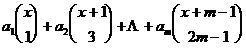where is an integer .A 3 ° integer-valued even polynomial ( satisfying f ( - x ) = f ( x )) must be expressed as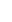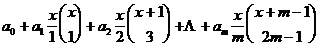where is an integer .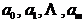[ Reducible and irreducible polynomials ]   Let f ( x ) be a polynomial with rational coefficients , if there are non-constant polynomials with rational coefficients g ( x ) and h ( x ), such that

f ( x ) = g ( x ) h ( x )

Then f ( x ) is called reducible ( or reducible) in the field of rational numbers , otherwise f ( x ) is called an irreducible polynomial over the field of rational numbers ( abbreviated as irreducible polynomial ).

[ Gauss's theorem ]   Let f ( x ) be an integer coefficient polynomial , which is reducible in the rational number field , then there must be two integer coefficient polynomials g ( x ) and h ( x ), such that

f ( x ) = g ( x ) h ( x )

[ Eisenstein's test ]

1 ° is set as . If there is a prime number p , such that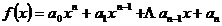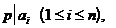but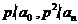Then f ( x ) is an irreducible polynomial .

2 ° is set as a polynomial of degree 2n+ 1 integer coefficient , if there is a prime number p , such that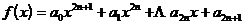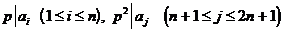butThen f ( x ) is an irreducible polynomial .

[ Pylon's Discrimination Method ]

1 ° setis an integer coefficient polynomial of degree n with a coefficient of 1 , which satisfies the conditions :

(i)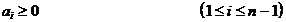(ii)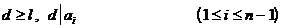(iii)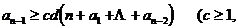Real numbers )

Then f ( x ) is an irreducible polynomial .

2 ° set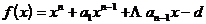is an integer coefficient polynomial of degree n with a coefficient of 1 , which satisfies the conditions :

(i)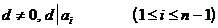(ii)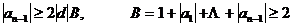Then f ( x ) is an irreducible polynomial .

3 ° setis an integer coefficient polynomial of degree n with a coefficient of 1 , which satisfies the conditions :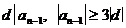Then f ( x ) is an irreducible polynomial .

4 ° set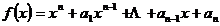is an integer coefficient polynomial of degree n with a coefficient of 1 , which satisfies the conditions :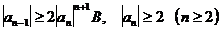Then f ( x ) is an irreducible polynomial .

5 ° setis an integer coefficient polynomial of degree n whose first term is 1 and whose constant term is not zero , and satisfies the conditions :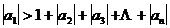Then f ( x ) is an irreducible polynomial .

[ Divisibility of Polynomials ]   Let f ( x ) and g ( x ) be polynomials with two rational coefficients , and g ( x ) is not always zero , if there is a polynomial h ( x ) such that

f ( x ) = g ( x ) h ( x )

Then g ( x ) is said to be divisible by f ( x ), denoted as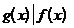orAt this time , g ( x ) is called a factor of f ( x ) , and f ( x ) is called a multiple of g ( x ) . Otherwise , g ( x ) cannot divide f ( x ), which is written as .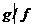The following ° f represents the degree of the polynomial f ( x ) .

The divisibility of polynomials has the following properties :

1 °2 ° If and , then f and g differ only by a constant factor .3 ° If , then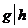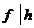4 ° if , then¶ ° f ° g _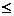If , and , then f is called a true factor of g , obviously ° f < ° g.5 ° If p ( x ) is an irreducible polynomial , and , then or .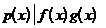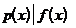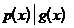6 ° If p ( x ) is an irreducible polynomial , and

f ( x ) = 0, p ( x ) = 0

If there is a common root , there must be .[ Polynomial with remainder division ]   Let f ( x ) and g ( x ) be arbitrary polynomials , and g ( x ) is not always zero , then there must be two polynomials q ( x ) and r ( x ) such that

f ( x ) = g ( x ) q ( x ) +r ( x )

where r ( x ) = 0 or ° r< ° g. This is called polynomial division with remainder .

[ Polynomial rolling division method ] The definition   of polynomial rolling and rolling division method and integer rolling division method are completely similar , and it is only necessary to regard the literal symbols in the formula in § 1(1) of this chapter as polynomials .

Similarly , the unique decomposition theorem of polynomials , the highest common factor and the lowest common multiple, and the concepts and formulas of polynomial coprime are completely similar to those in the section on integers , and it is only necessary to regard the symbols in the corresponding formulas as polynomials .

Example Polynomial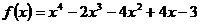and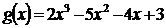The highest common factor of .

Solution To avoid fractions , multiply f ( x ) by 2 , then divide f ( x ) by g ( x ) :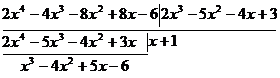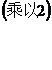During the calculation , the first difference is multiplied by 2 , so the quotient is changed , but the remainder only obtains a number factor of 2, which does not matter . Multiply g ( x ) by 3 , and divide by :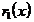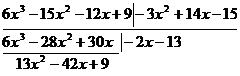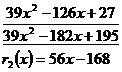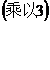desirable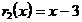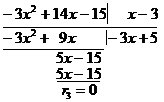So the required common factor is .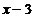[ Congruence ]

1 ° polynomial mode congruence Let m ( x ) be a polynomial , if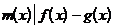Then f ( x ) and g ( x ) are said to be congruent modulo m ( x ) , denoted as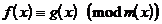2 ° Prime Modulo Congruence Let p be a prime number , f ( x ) and g ( x ) are polynomials with integer coefficients , if the corresponding coefficients are all congruent modulo p , then the two polynomials are said to be congruent modulo p , and write do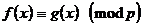3 ° Multiple modular congruence formula Let p be a prime number , ( x ) is a polynomial , if f ( x ) - g ( x ) is a multiple of ( x ) , mod p , then f ( x ) and g ( x ) Congruence modulo p, ( x ) , denoted as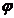f ( x ) g ( x )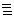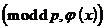[ Promotion of Fermat's Theorem ]   Let p be a prime number , ( x )is an irreducible polynomial of degree n , mod p, then for any polynomial that is not a multiple of ( x ) f ( x ), mod p, there is always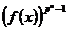1For any polynomial there is always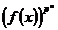f ( x )veryx